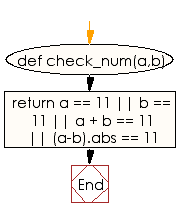﻿ Ruby Basic exercises: Check two given integers and return true if either one is 11 or their sum or difference is 11 otherwise return false - w3resource# Ruby Basic Exercises: Check two given integers and return true if either one is 11 or their sum or difference is 11 otherwise return false

## Ruby Basic: Exercise-45 with Solution

Write a Ruby program to check two given integers and return true if either one is 11 or their sum or difference is 11 otherwise return false.

Ruby Code:

``````def check_num(a,b)
return a == 11 || b == 11 || a + b == 11 || (a-b).abs == 11
end

print check_num(9, 11),"\n"
print check_num(13, 7),"\n"
print check_num(22, 11),"\n"
print check_num(21, 32),"\n"
print check_num(20, 30)
``````

Output:

```true
false
true
true
false
```

Flowchart:Ruby Code Editor: